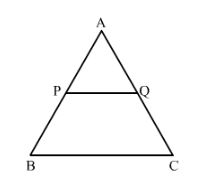# In ∆ABC, PQ is a line segment intersecting AB at P and AC at Q such

Question:

In ∆ABC, PQ is a line segment intersecting AB at P and AC at Q such that PQ || BC and PQ divides ∆ABC into two parts equal in area. Find BPAB.

Solution:

Given: In ΔABC, PQ is a line segment intersecting AB at P, and AC at Q such that PQ || BC and PQ divides ΔABC in two parts equal in area.

To find: $\frac{\mathrm{BP}}{\mathrm{AB}}$

We have PQ $\|$ BC

And

$A r(\triangle \mathrm{APQ})=A r($ quad $\mathrm{BPQC})$

$\operatorname{Ar}(\triangle \mathrm{APQ})+\operatorname{Ar}(\triangle \mathrm{APQ})=\operatorname{Ar}($ quad BPQC $)+\operatorname{Ar}(\triangle \mathrm{APQ})$

$2 A r(\triangle \mathrm{APQ})=A r(\triangle \mathrm{ABC})$.....(1)

Now, PQ || BC and BA is a transversal.

In ΔAPQ and ΔABC,

∠APQ=∠B              Corresponding angles

∠PAQ=∠BAC       Common

So, ∆APQ~∆ABC      (AA Similarity)We know that the ratio of areas of two similar triangles is equal to the ratio of squares of their corresponding sides.

Hence

$\frac{A r(\Delta \mathrm{APQ})}{A r(\Delta \mathrm{ABC})}=\frac{\mathrm{AP}^{2}}{\mathrm{AB}^{2}}$

$\frac{A r(\Delta \mathrm{APQ})}{2 A r(\Delta \mathrm{APQ})}=\frac{\mathrm{AP}^{2}}{\mathrm{AB}^{2}}$

$\frac{1}{2}=\frac{\mathrm{AP}^{2}}{\mathrm{AB}^{2}}$

$\sqrt{\frac{1}{2}}=\frac{\mathrm{AP}}{\mathrm{AB}}$

$A B=\sqrt{2} A P$

$\mathrm{AB}=\sqrt{2}(\mathrm{AB}-\mathrm{BP})$

$\sqrt{2} \mathrm{BP}=\sqrt{2} \mathrm{AB}-\mathrm{AB}$

$\sqrt{2} \mathrm{BP}=(\sqrt{2}-1) \mathrm{AB}$

$\frac{\mathrm{BP}}{\mathrm{AB}}=\frac{(\sqrt{2}-1)}{\sqrt{2}}$